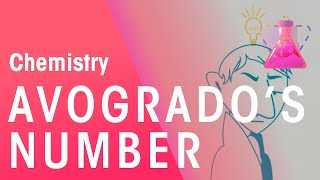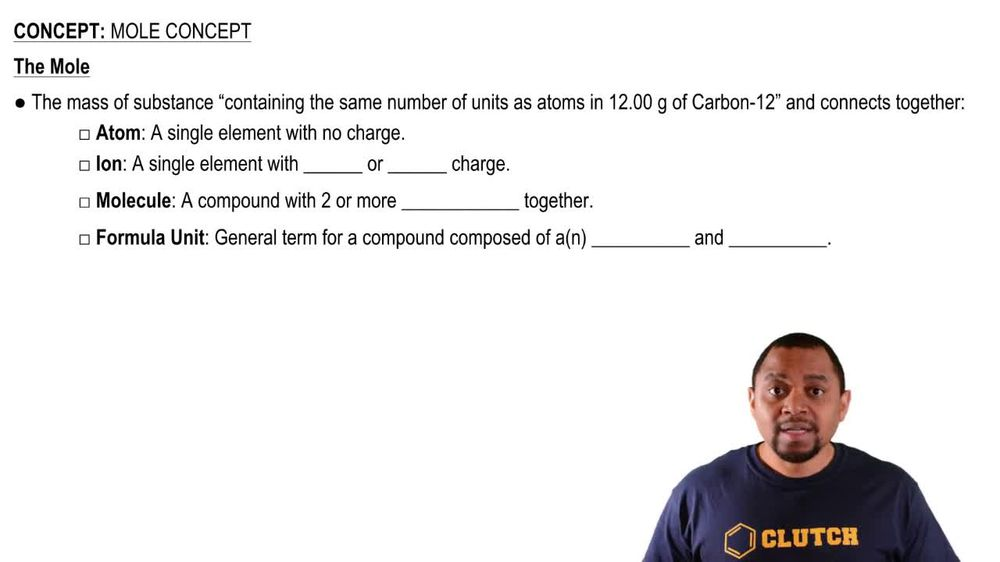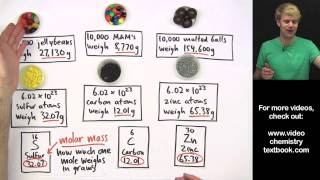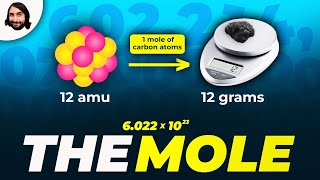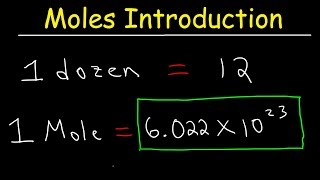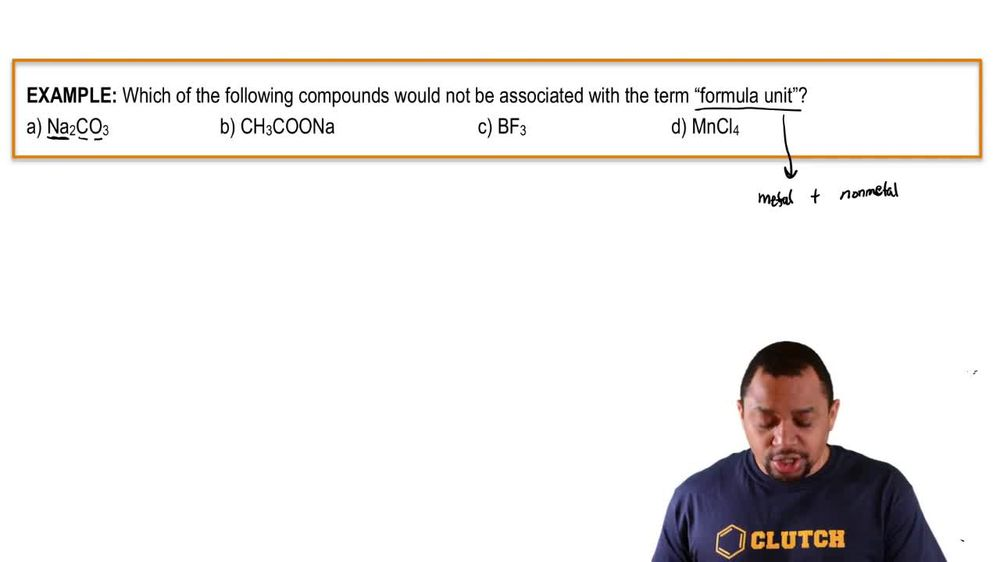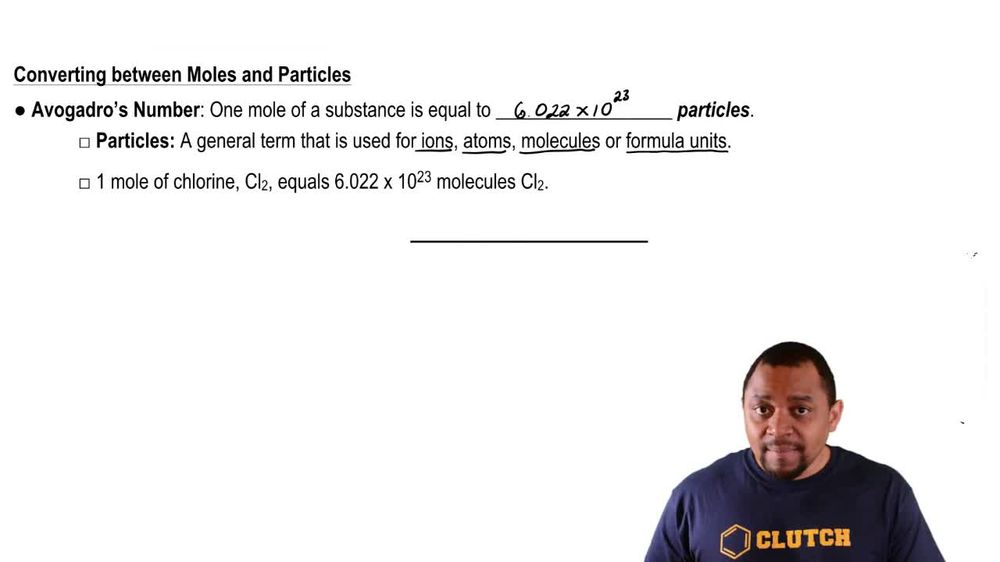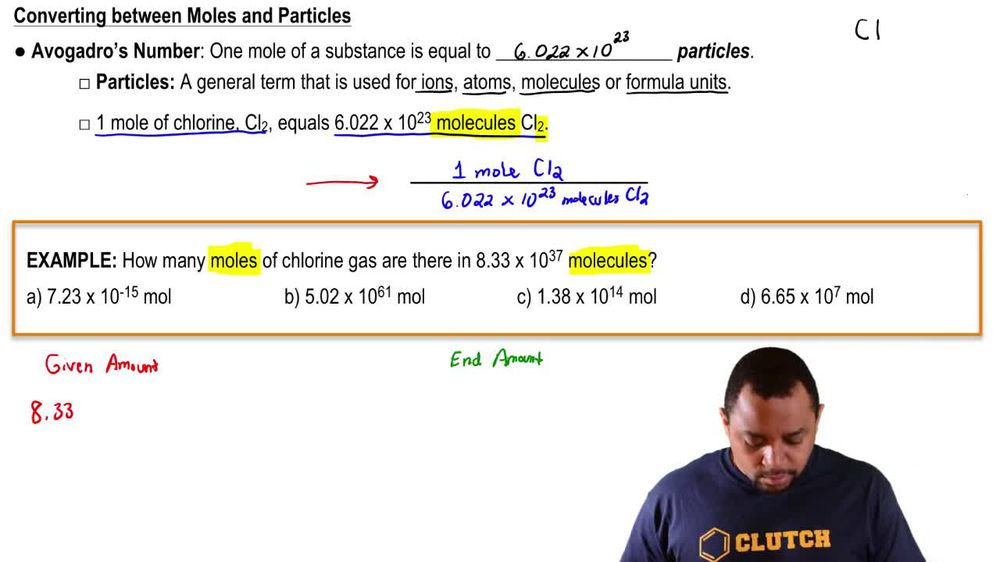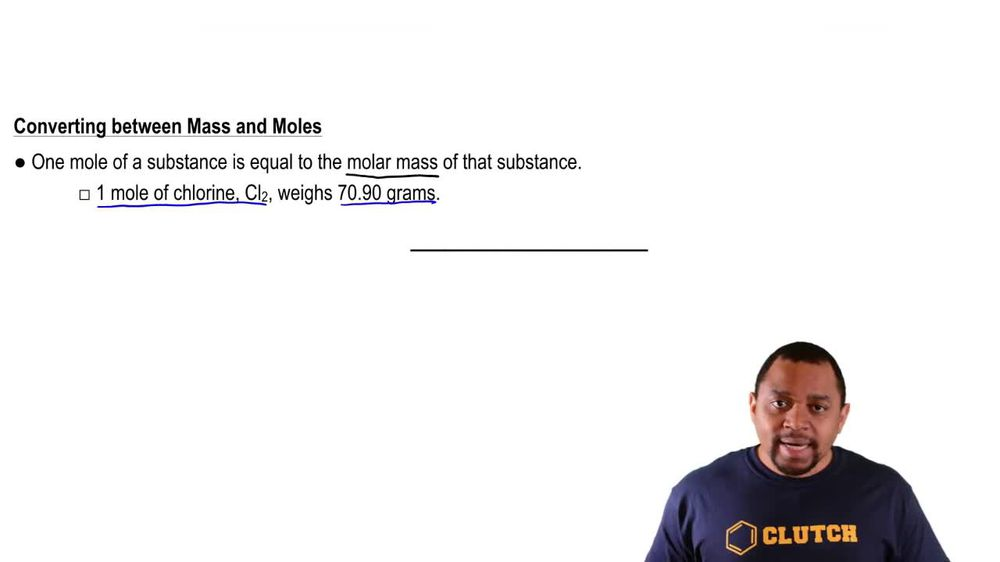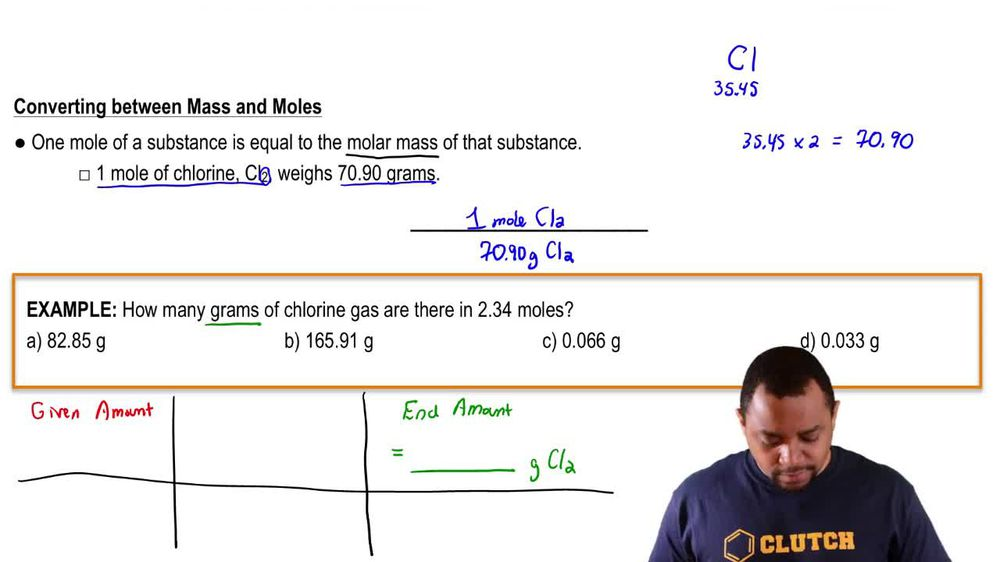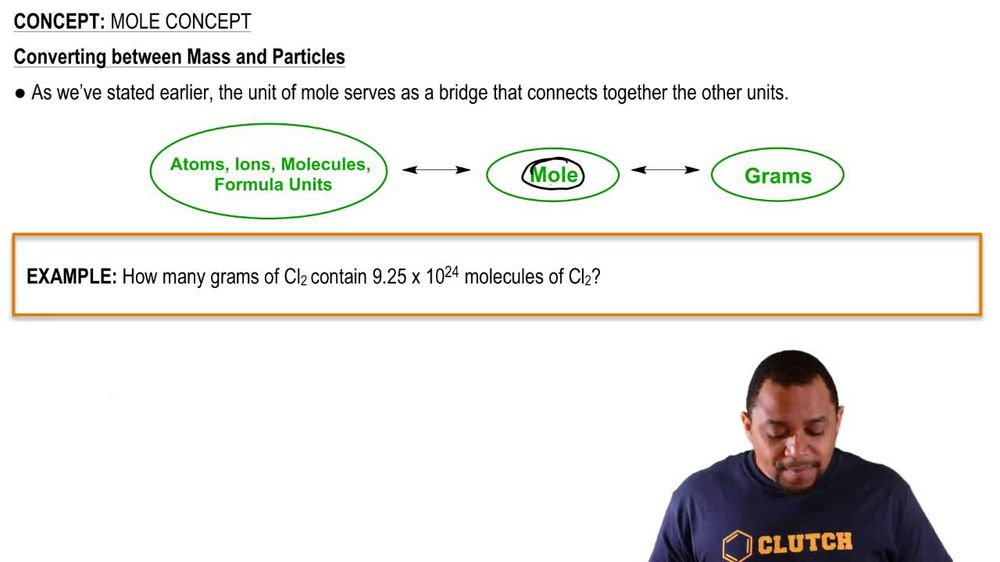Start typing, then use the up and down arrows to select an option from the list.
1. 2. Atoms & Elements2. Mole Concept
Problem

# The molecular formula of salicylic acid, a compound commonly found in facial cleanser, is C7H6O3. (c) How many molecules of salicylic acid are in 0.5 mg of this substance?

Relevant Solution2m
Play a video:
Hi everyone Welcome back. So let's get started with this video. So here they want us to calculate the number of moles of ammonium ions And 8.963 g of ammonium sulfate. Okay, so then ammonium sulfite is composed of NH four One plus which is ammonium. And then Sophie is S two so the compound could have a charge of zero. So then and age four, two and so forth. So this is ammonium Sophie. Okay. And then we're also starting off with grams because that is what is given. And then from grams we're going to go to moles and how are we going to go from grams to moles. We're going to use a molar mass of ammonium Sophie And we find it to be 100 32 point 14 Graham's promote Okay, and then for moles we're going to find the most of ammonium ions. So let's go ahead and get started with our Sochi aama tree. So let's start with the grounds given, which is eight point g Mm Ammonium sulfate. So the NH four, two s 04 and then we're going to use the mortar, Mastic little moles Which he said is .14g of ammonium sulfate for one more of ammonium Sophie and then we're going to use moles to find the most of any HR contained. So then one more Good morning. Sophie contains two malls of ammonium ions. So then grams of ammonium sulfate canceled out. Most of ammonium sulfate cancel out. And we get malls of ammonium ions. We do the math and we get zero point 1357 most of ammonium iron. Okay, thank you for watching. I hope this helped. And I'll see you in the next video.